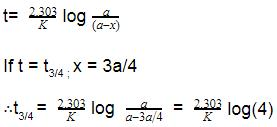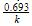Courses

# Test: Integrated Rate Equation

## 10 Questions MCQ Test Chemistry for JEE | Test: Integrated Rate Equation

Description
This mock test of Test: Integrated Rate Equation for Class 12 helps you for every Class 12 entrance exam. This contains 10 Multiple Choice Questions for Class 12 Test: Integrated Rate Equation (mcq) to study with solutions a complete question bank. The solved questions answers in this Test: Integrated Rate Equation quiz give you a good mix of easy questions and tough questions. Class 12 students definitely take this Test: Integrated Rate Equation exercise for a better result in the exam. You can find other Test: Integrated Rate Equation extra questions, long questions & short questions for Class 12 on EduRev as well by searching above.
QUESTION: 1

### The half life of a first order reaction is equal to:

Solution:

The correct answer is Option C
For a first-order reaction, the half-life is given by: t1/2 = 0.693/k

QUESTION: 2

### For zero order reaction, linear plot was obtained for [A] vs t, the slope of the line is equal to:

Solution:

For zero order reaction the rate equation is [A] = -kt + [Ao]

Thus the slope of the line is -ko

QUESTION: 3

### The first order rate constant for the decomposition of N2O5 is 6.2 x 10-4 sec-1. The t1/2 of the decomposition reaction is

Solution:

The correct answer is option  B
t1/2​ = k0.693​
= 0.693​/(6.2 × 10− 4)​ = 1117.7s

QUESTION: 4

Find the overall order of a reaction whose rate constant is k = 3x10-4 s-1

Solution:

The correct answer is option D
Units:- for K can be calculated by (Mole)1-n (Litre )n-1 Time -1...
Here,n is the order of the reaction ....
On substituting n = 1.
We get, K units as;
K = (Mole)1-1( Litre)1-1 sec-1.
K=sec-1.......

QUESTION: 5

Which of the following represents the expression for the 3/4th life of a first order reaction?

Solution:

The correct answer is option A
As we know, for a first order reaction,QUESTION: 6

The type of reaction that gives constant half life is

Solution:

The correct answer is Option A
For first order reaction, the half-life period is independent of initial concentration.
For first order reaction, the half-life period expression (t1/2) is given by the expression t1/2=, where k is the rate constant.

QUESTION: 7

The half life period of first order reaction is 15 min. Its rate constant will be equal to

Solution:

The half life of a reaction is 15 min
So,
Here we go..
Formula to be used :

K =0.693/t = 0.693/15
__= 0.462/10 = 0.0462 min^-1

QUESTION: 8

For zero order reaction, the integrated rate equation is:

Solution:
QUESTION: 9

The half life of a zero order reaction is equal to:

Solution:

[A] = -kt + [A]0
Here, [A] = [A0]/2
[A]0/2 = -kt + [A]0
kt = [A]0 - [A]0/2
t = [A]0/2k
t1/2 = [A]0/2k

QUESTION: 10

If [A] is the concentration of reactant A at any time t and [Ao] is the concentration at t=0, then for the first order reaction the rate equation can be written as:

Solution: